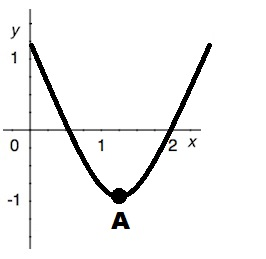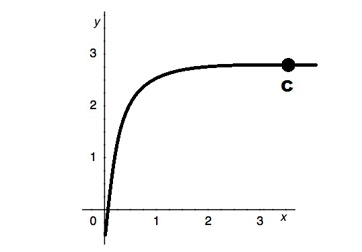# Graphing the Derivative from Any Function - Quiz & Worksheet

Instructions:

question 1 of 3

### The derivative of the following graph is _____ at point A.Create Your Account To Take This Quiz

As a member, you'll also get unlimited access to over 84,000 lessons in math, English, science, history, and more. Plus, get practice tests, quizzes, and personalized coaching to help you succeed.

Try it risk-free for 30 days. Cancel anytime

### 2. The derivative of the following graph is _____ at point C.Create your account to access this entire worksheet
Quizzes, practice exams & worksheets
Certificate of Completion
Create an account to get started

This quiz and corresponding worksheet will help you gauge your knowledge of how to graph the derivative from any function by presenting you with a series of problems in which you'll need to identify the derivative.

## Quiz & Worksheet Goals

Use this printable worksheet and quiz to:

• Identify the derivative of a graph
• Determine the graph of the derivative of a given function

## Skills Practiced

This worksheet and quiz will let you practice the following skills:

• Knowledge application - access your knowledge to identify the respective derivatives of several graphs
• Problem solving - use acquired knowledge to solve various graphing the derivative problems
• Reading comprehension - ensure that you have understood the most important information from the related calculus lesson

For more on this subject, review the corresponding lesson titled Graphing the Derivative from Any Function. This lesson will help you:

• Understand the rules of derivation
• Describe how location can serve as a function of time
• Identify the derivatives of a function
• Appreciate the nature of discontinuous derivatives
• Show how to find the derivative of a single location
Final ExamMath 104: Calculus
Status: Not Started
Chapter ExamGraphing Derivatives and L'Hopital's Rule
Status: Not Started

Support